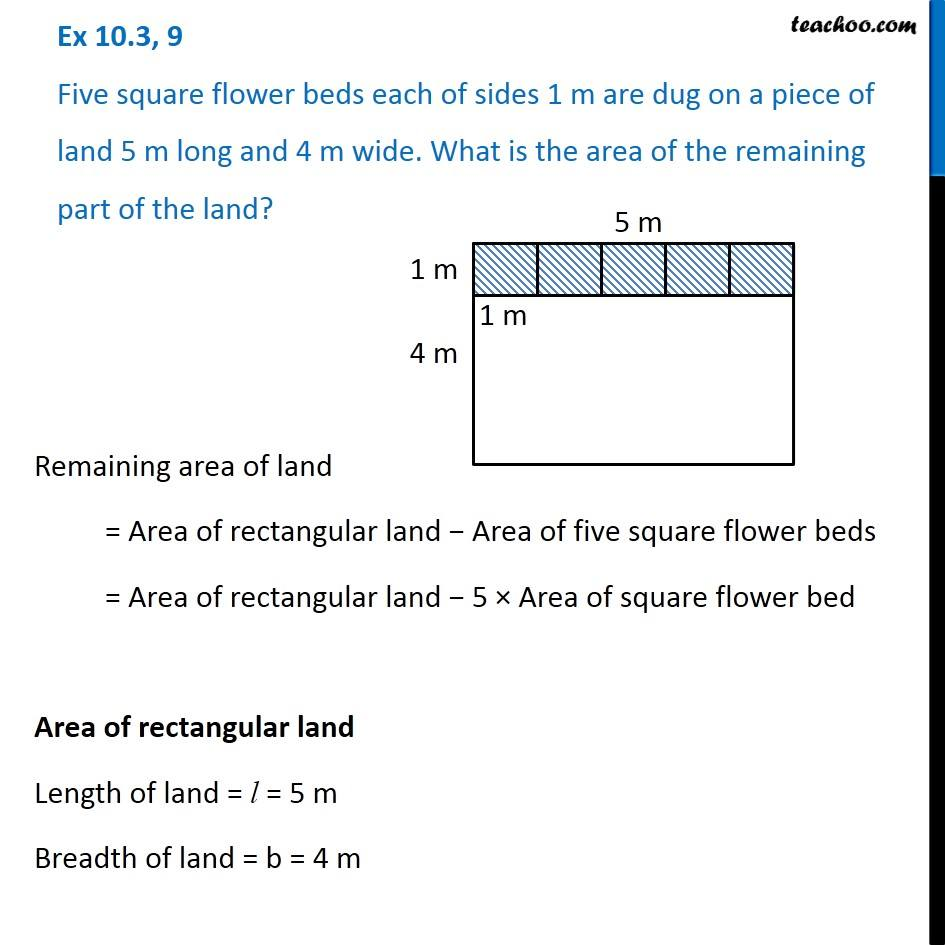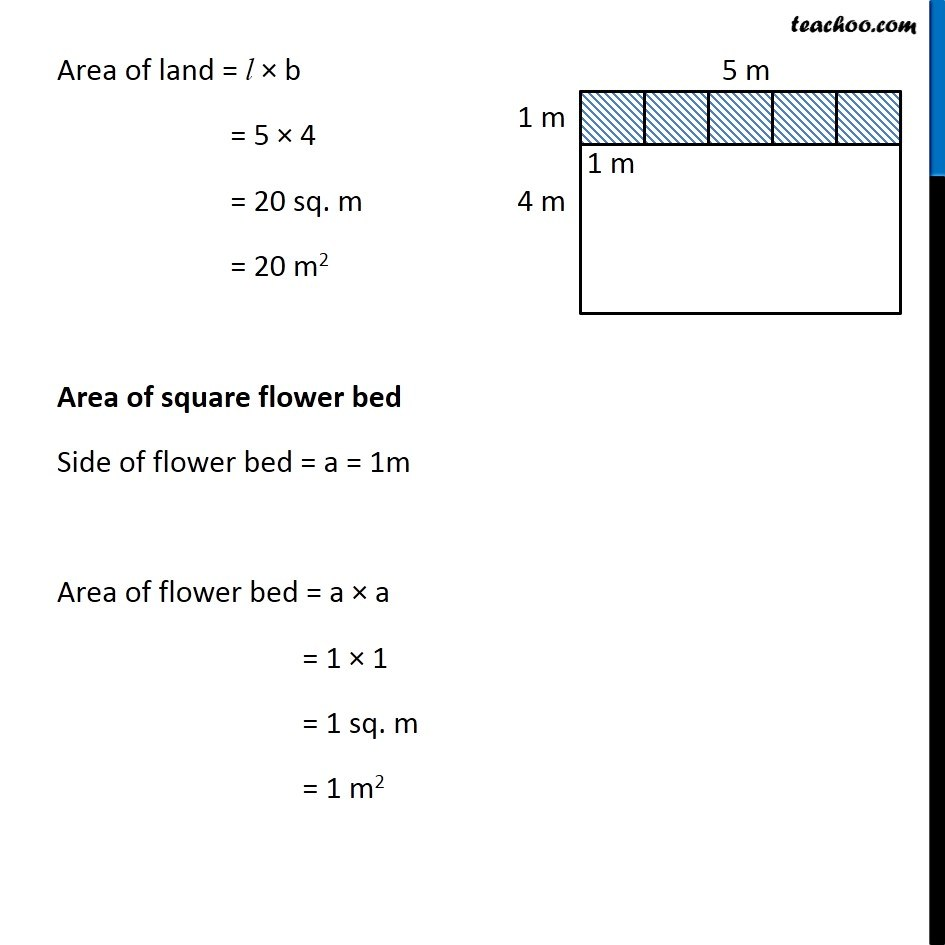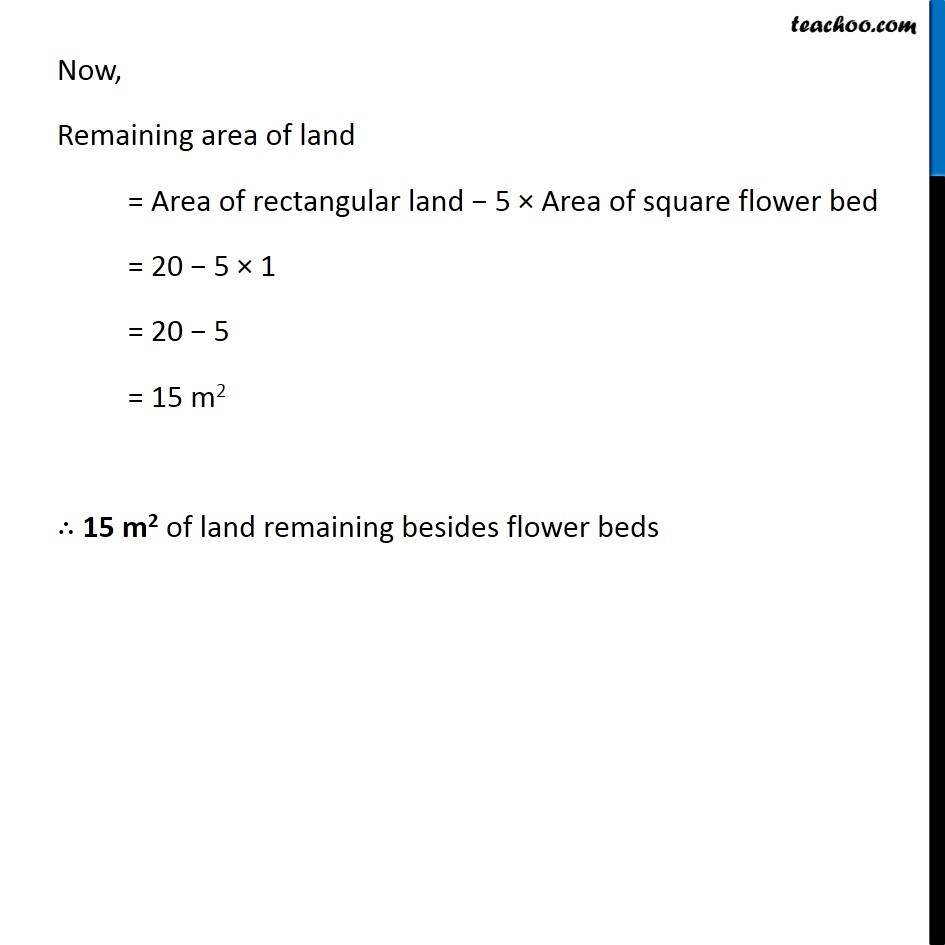Ex 10.3

Chapter 10 Class 6 Mensuration
Serial order wiseLearn in your speed, with individual attention - Teachoo Maths 1-on-1 Class

### Transcript

Ex 10.3, 9 Five square flower beds each of sides 1 m are dug on a piece of land 5 m long and 4 m wide. What is the area of the remaining part of the land? Remaining area of land = Area of rectangular land − Area of five square flower beds = Area of rectangular land − 5 × Area of square flower bed Area of rectangular land Length of land = l = 5 m Breadth of land = b = 4 m Area of land = l × b = 5 × 4 = 20 sq. m = 20 m2 Area of square flower bed Side of flower bed = a = 1m Area of flower bed = a × a = 1 × 1 = 1 sq. m = 1 m2 Now, Remaining area of land = Area of rectangular land − 5 × Area of square flower bed = 20 − 5 × 1 = 20 − 5 = 15 m2 ∴ 15 m2 of land remaining besides flower beds